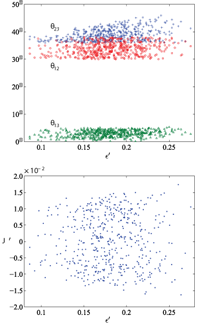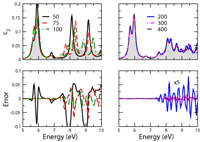February 2011
Mon Tue Wed Thu Fri Sat Sun
« Jan   Mar »
123456
78910111213
14151617181920
21222324252627
28

## Non-Hermitian Perturbations to the Fritzsch Textures of Lepton and Quark Mass Matrices

Harald Fritzsch, Zhi-zhong Xing, Ye-Ling ZhouWe show that non-Hermitian and nearest-neighbor-interacting perturbations to the Fritzsch textures of lepton and quark mass matrices can make both of them fit current experimental data very well. In particular, we obtain \theta_{23} \simeq 45^\circ for the atmospheric neutrino mixing angle and predict \theta_{13} \simeq 3^\circ to 6^\circ for the smallest neutrino mixing angle when the perturbations in the lepton sector are at the 20% level. The same level of perturbations is required in the quark sector, where the Jarlskog invariant of CP violation is about 3.7 \times 10^{-5}. In comparison, the strength of leptonic CP violation is possible to reach about 1.5 \times 10^{-2} in neutrino oscillations.

http://arxiv.org/abs/1101.4272
High Energy Physics – Phenomenology (hep-ph)

## Implementation and testing of Lanczos-based algorithms for Random-Phase Approximation eigenproblems

Myrta Grüning, Andrea Marini, Xavier GonzeThe treatment of the Random-Phase Approximation Hamiltonians, encountered in different frameworks, like Time-Dependent Density Functional Theory or Bethe-Salpeter equation, is complicated by their non-Hermicity. Compared to their Hermitian Hamiltonian counterparts, computational methods for the treatment of non-Hermitian Hamiltonians are often less efficient and less stable, sometimes leading to the breakdown of the method. Recently [Gruning et al. Nano Lett. 8, 2820 (2009)], we have identified that such Hamiltonians are usually pseudo-Hermitian. Exploiting this property, we have implemented an algorithm of the Lanczos type for random-Phase Approximation Hamiltonians that benefits from the same stability and computational load as its Hermitian counterpart, and applied it to the study of the optical response of carbon nanotubes. We present here the related theoretical grounds and technical details, and study the performance of the algorithm for the calculation of the optical absorption of a molecule within the Bethe-Salpeter equation framework.

http://arxiv.org/abs/1102.3909
Materials Science (cond-mat.mtrl-sci); Mathematical Physics (math-ph)